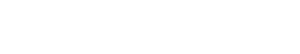# Mean

Mean is a measure of central tendency. Central tendency is a way to describe where most of the data lies in a distribution. The three most common measures of central tendency are mean, median, and mode.

## Importance of Identifying the Mean

Text analytics is the process of turning unstructured text into meaningful data for analysis, to gain insights into trends, behaviors, and opinions. One of the most important steps in text analytics is identifying the mean. The mean is used to determine the overall sentiment of a document or collection of documents. It can also be used to identify key topics in a document or collection of documents.

## Mean Calculation

Mean is the average of all the values in a distribution. To calculate the mean, you add up all the values in a data set and then divide by the number of values in the data set.

For example, let’s say you have the following data set:

1, 2, 3, 4, 5

To calculate the mean of this data set, you would add up all the values (1 + 2 + 3 + 4 + 5) and then divide by the number of values in the data set (5). This would give you a mean of 3.Unlock the power of actionable insights with AI-based natural language processing.